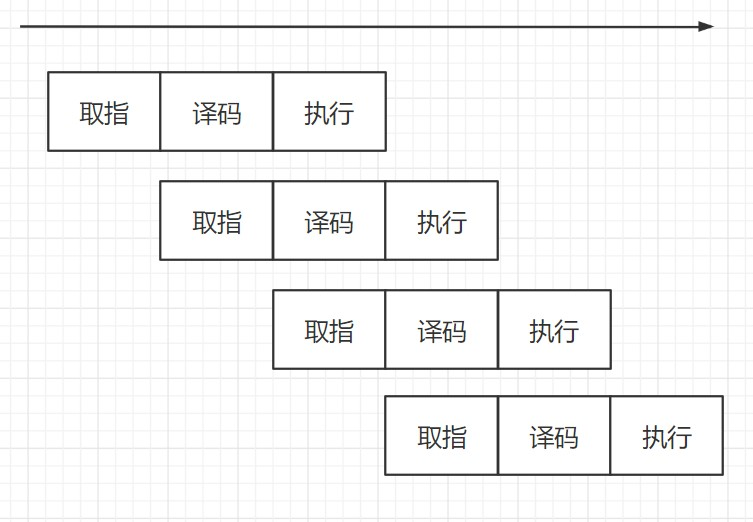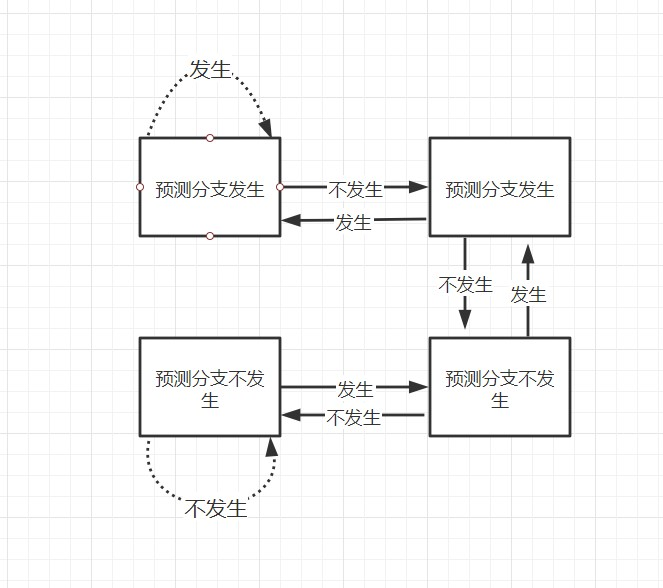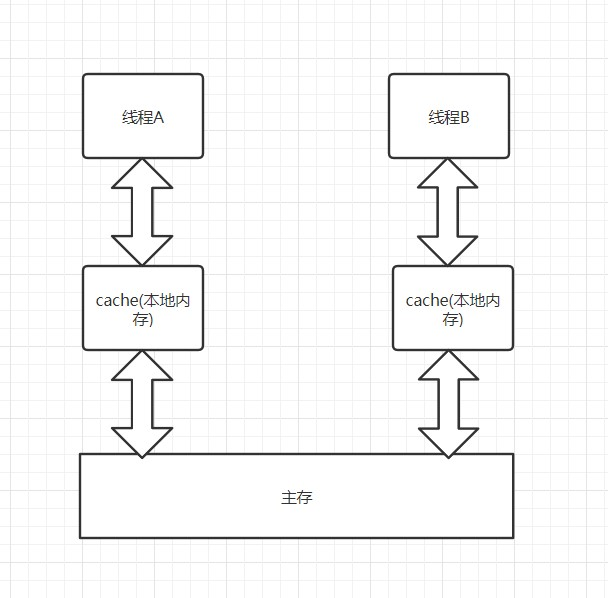﻿ 学习计算机组成原理有什么用，意义何在？-编程-比特在线

# 学习计算机组成原理有什么用？

## 从StackOverFlow上一个很经典的问题说起

``````import java.util.Arrays;
import java.util.Random;

public class Test
{
public static void main(String[] args)
{
// Generate data
int arraySize = 32768;
int data[] = new int[arraySize];

Random rnd = new Random(0);
for (int c = 0; c < arraySize; ++c)
data[c] = rnd.nextInt() % 256;

// !!! With this, the next loop runs faster
//Arrays.sort(data);

// Test
long start = System.nanoTime();
long sum = 0;

for (int i = 0; i < 100000; ++i)
{
// Primary loop
for (int c = 0; c < arraySize; ++c)
{
if(data[c]>128)
sum+=data[c];
}
}

System.out.println((System.nanoTime() - start) / 1000000000.0);
System.out.println("sum = " + sum);
}
}

``````

`````` 			   int t = (data[c] - 128) >> 31;
sum += ~t & data[c];
``````

## 为了快点写完作业，我选择瞎蒙``````                if(data[c]>128)
sum+=data[c];
``````

## 好像和我程序里写的不一样

``````public class Thread01 implements Runnable {
private int cnt=10;

@Override
public void run() {
while (cnt>0){
try {
} catch (InterruptedException e) {
e.printStackTrace();
}
}
}

public static void main(String[] args) {
a.start();
b.start();
c.start();
d.start();
e.start();
}
}

``````